# NCERT Solutions for Class 11 Physics chapter-6 Work, Energy And Power

## NCERT Solutions for Class 11 Physics

NCERT Solutions for class-11 Physics Chapter-6 Work, Energy and Power is prepared by our senior and renowned teachers of pw primary focus while solving these questions of class-11 in NCERT textbook, also do read theory of this Chapter-6 Work, Energy and Power while going before solving the NCERT questions. You can download NCERT solution of all chapters from Physics Wallah in PDF.

### Chapter-6 Work, Energy and Power

Question1. The sign of work done by a force on a body is important to understand. State carefully if the following quantities are positive or negative:
a. work done by a man in lifting a bucket out of a well by means of a rope tied to the bucket.
b. work done by gravitational force in the above case,
c. work done by friction on a body sliding down an inclined plane,
d. work done by an applied force on a body moving on a rough horizontal plane with uniform velocity,
e. work done by the resistive force of air on a vibrating pendulum in bringing it to rest.

Solution :
a. Positive
In the given case, force and displacement are in the same direction. Hence, the sign of work done is positive. In this case, the work is done on the bucket.

b. Negative
In the given case, the direction of force (vertically downward) and displacement (vertically upward) are opposite to each other. Hence, the sign of work done is negative.

c. Negative
Since the direction of frictional force is opposite to the direction of motion, the work done by frictional force is negative in this case.

d. Positive
Here the body is moving on a rough horizontal plane. Frictional force opposes the motion of the body. Therefore, in order to maintain a uniform velocity, a uniform force must be applied to the body. Since the applied force acts in the direction of motion of the body, the work done is positive.

e. Negative
The resistive force of air acts in the direction opposite to the direction of motion of the pendulum. Hence, the work done is negative in this case.

Question2. A body of mass 2 kg initially at rest moves under the action of an applied horizontal force of 7 N on a table with coefficient of kinetic friction = 0.1.
Compute the

a. work done by the applied force in 10 s,
b. work done by friction in 10 s,
c. work done by the net force on the body in 10 s,
d. change in kinetic energy of the body in 10 s,

Solution :
Mass of the body, m = 2 kg Applied force, F = 7 N
Coefficient of kinetic friction, µ = 0.1
Initial velocity, u = 0
Time, t = 10 s
The acceleration produced in the body by the applied force is given by Newton’s second law of motion as:
a' = F / m = 7 / 2 = 3.5 ms-2
Frictional force is given as:
f = µmg
= 0.1 × 2 × 9.8 = – 1.96 N
The acceleration produced by the frictional force:
a" = -1.96 / 2 = -0.98 ms-2
Total acceleration of the body: a' + a"
= 3.5  + (-0.98) = 2.52 ms-2
The distance travelled by the body is given by the equation of motion:
s = ut + (1/2)at2
= 0 + (1/2)  ×  2.52  ×  (10)2 = 126 m

a. Work done by the applied force, Wa = F  × s  = 7 × 126 = 882 J
b. Work done by the frictional force, Wf = F  × s  = –1.96  × 126 = –247 J
c. Net force = 7 + (–1.96) = 5.04 N
Work done by the net force, Wnet= 5.04 × 126 = 635 J
d. From the first equation of motion, final velocity can be calculated as:
v = u + at
= 0 + 2.52  × 10 = 25.2 m/s
Change in kinetic energy = (1/2) mv2 - (1/2) mu2
= (1/2)  × 2 (v2 - u2) = (25.2)2 - 02 = 635 J

Question3. Given in Fig. 6.11 are examples of some potential energy functions in one dimension. The total energy of the particle is indicated by a cross on the ordinate axis. In each case, specify the regions, if any, in which the particle cannot be found for the given energy. Also, indicate the minimum total energy the particle must have in each case. Think of simple physical contexts for which these potential energy shapes are relevant.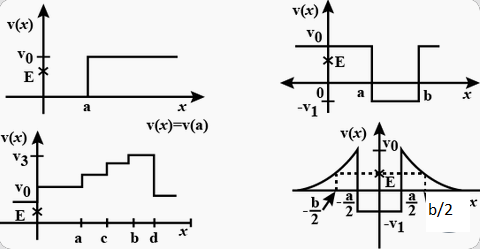Solution :
Total energy of a system is given by the relation:
E = P.E. + K. E.
∴ K.E. = E – P.E.
Kinetic energy of a body is a positive quantity. It cannot be negative. Therefore, the particle will not exist in a region where K.E. becomes negative.
a. For x > a, P.E. (V0) > E ∴ K.E. becomes negative. Hence, the object cannot exist in the region x > a.
b.  For x < a and x > b, P.E. (V0) > E. ∴ K.E. becomes negative. Hence the object cannot be present in the region x < a and x > b.
c.  x > a and x < b;  –V1 In the given case, the condition regarding the positivity of K.E. is satisfied only in the region between x > a and x < b.
The minimum potential energy in this case is –V1. Therfore, K.E.  = E – (–V1) = E + V1. Therefore, for the positivity of the kinetic energy, the total energy of the particle must be greater than –V1. So, the minimum total energy the particle must have is –V1.

d.  -b/2 <  x <  a/2 ; a/2 < x < b/2 ; -V1

In the given case, the potential energy (V0) of the particle becomes greater than the total energy (E) for -b/2 < x < b/2 and -a/2 <  x < a/2. Therefore, the particle will not exist in these regions.
The minimum potential energy in this case is –V1. Therfore, K.E.  = E – (–V1) = E + V1. Therefore, for the positivity of the kinetic energy, the totaol energy of the particle must be greater than –V1. So, the minimum total energy the particle must have is –V1.

Question4. The potential energy function for a particle executing linear simple harmonic motion is given by V(x) =kx2/2, where k is the force constant of the oscillator. For k = 0.5 N m–1, the graph of V(x) versus x is shown in Fig. 6.12. Show that a particle of total energy 1 J moving under this potential must ‘turn back’ when it reaches x = ± 2 m.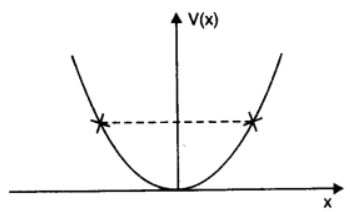Solution :
Total energy of the particle, E = 1 J Force constant, k = 0.5 N m–1
Kinetic energy of the particle, K = (1/2)mv2
According to the conservation law:
E = V + K
1 = (1/2) kx2 + (1/2)mv2
At the moment of ‘turn back’, velocity (and hence K) becomes zero.
∴ 1 = (1/2) kx2
(1/2)  × 0.5 x2 = 1
x2 = 4
x = ±2
Hence, the particle turns back when it reaches x = ± 2 m.

a. The casing of a rocket in flight burns up due to friction. At whose expense is the heat energy required for burning obtained? The rocket or the atmosphere?
b. Comets move around the sun in highly elliptical orbits. The gravitational force on the comet due to the sun is not normal to the comet’s velocity in general. Yet the work done by the gravitational force over every complete orbit of the comet is zero. Why?
c. An artificial satellite orbiting the earth in very thin atmosphere loses its energy gradually due to dissipation against atmospheric resistance, however small. Why then does its speed increase progressively as it comes closer and closer to the earth?
d. In Fig. 6.13(i) the man walks 2 m carrying a mass of 15 kg on his hands. In Fig. 6.13(ii), he walks the same distance pulling the rope behind him. The rope goes over a pulley, and a mass of 15 kg hangs at its other end. In which case is the work done greater?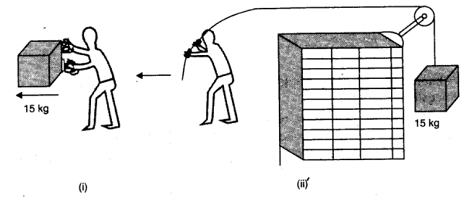Solution :

a. Rocket The burning of the casing of a rocket in flight (due to friction) results in the reduction of the mass of the rocket.
According to the conservation of energy:
Total energy = Potential energy + Kinetic energy
= mgh + (1/2)mv2
The reduction in the rocket’s mass causes a drop in the total energy. Therefore, the heat energy required for the burning is obtained from the rocket.

b. Gravitational force is a conservative force. Since the work done by a conservative force over a closed path is zero, the work done by the gravitational force over every complete orbit of a comet is zero.

c. When an artificial satellite, orbiting around earth, moves closer to earth, its potential energy decreases because of the reduction in the height. Since the total energy of the system remains constant, the reduction in P.E. results in an increase in K.E. Hence, the velocity of the satellite increases. However, due to atmospheric friction, the total energy of the satellite decreases by a small amount.

d.  Work done in fig 6.13 (i)
Mass, m = 15 kg
Displacement, s = 2 m
Work done, W = Fs Cosθ
Where, θ = Angle between force and displacement
= mgs Cosθ = 15  × 2  × 9.8 Cos 900
= 0
Work done in fig 6.13 (ii)
Mass, m = 15 kg
Displacement, s = 2 m
Here, the direction of the force applied on the rope and the direction of the displacement of the rope are same.
Therefore, the angle between them, θ = 0°
Since cos 0° = 1
Work done, W = Fs cosθ = mgs
= 15 × 9.8 × 2 = 294 J

Hence, more work is done in the Fig. 6.13 (ii).

Question6. Underline the correct alternative:
a. When a conservative force does positive work on a body, the potential energy of the body increases /decreases /remains unaltered.
b. Work done by a body against friction always results in a loss of its kinetic /potential energy.
c. The rate of change of total momentum of a many-particle system is proportional to the external force/sum of the internal forces on the system.
d. In an inelastic collision of two bodies, the quantities which do not change after the collision are the total kinetic energy /total linear momentum/total energy of the system of two bodies.

Solution :
a. Decreases
A conservative force does a positive work on a body when it displaces the body in the direction of force. As a result, the body advances toward the centre of force. It decreases the separation between the two, thereby decreasing the potential energy of the body.

b. Kinetic energy
The work done against the direction of friction reduces the velocity of a body. Hence, there is a loss of kinetic energy of the body.

c. External force
Internal forces, irrespective of their direction, cannot produce any change in the total momentum of a body. Hence, the total momentum of a many- particle system is proportional to the external forces acting on the system.

d. Total linear momentum
The total linear momentum always remains conserved whether it is an elastic collision or an inelastic collision.

Question7. State if each of the following statements is true or false. Give reasons for your answer.
a.  In an elastic collision of two bodies, the momentum and energy of each body is conserved.
b.  Total energy of a system is always conserved, no matter what internal and external forces on the body are present.
c.  Work done in the motion of a body over a closed loop is zero for every force in nature.
d.  In an inelastic collision, the final kinetic energy is always less than the initial kinetic energy of the system.

Solution :
a.  False
In an elastic collision, the total energy and momentum of both the bodies, and not of each individual body, is conserved.

b.  False
The external forces on the body may cahnge the total energy of the body.

c.  False
The work done in the motion of a body over a closed loop is zero for a conservation force only.

d.  True
In an inelastic collision, the final kinetic energy is always less than the initial kinetic energy of the system. This is because in such collisions, there is always a loss of energy in the form of heat, sound, etc.

a.  In an elastic collision of two billiard balls, is the total kinetic energy conserved during the short time of collision of the balls (i.e. when they are in contact)?
b.  Is the total linear momentum conserved during the short time of an elastic collision of two balls?
c.  What are the answers to (a) and (b) for an inelastic collision?
d. If the potential energy of two billiard balls depends only on the separation distance between their centres, is the collision elastic or inelastic? (Note, we are talking here of potential energy corresponding to the force during collision, not gravitational potential energy).

Solution :
a. No
K.E. is not conserved during the given elastic collision, K.E. before and after collision is the same. Infact, during collision, K.E. of the balls gets converted into potential energy.

b. Yes
In an elastic collision, the total linear momentum of the system always remains conserved.

c. No; Yes
In an inelastic collision, there is always a loss of kinetic energy, i.e., the total kinetic energy of the billiard balls before collision will always be greater than that after collision.
The total linear momentum of the system of billiards balls will remain conserved even in the case of an inelastic collision.

d.  Elastic
In the given case, the forces involved are conservation. This is because they depend on the separation between the centres of the billiard balls. Hence, the collision is elastic.

Question9.A body is initially at rest. It undergoes one-dimensional motion with constant acceleration. The power delivered to it at time t is proportional to
a.  t1/2

b.  t

c.  t3/2

d.  t2

Solution :
From,
v = u + at
v = 0 + at = at
As power, P = F × v
∴ P = (ma) × at = ma2t
As m and a are constants, therefore, P ∝ t
Hence, right choice is (b) t

Question10. A body is moving unidirectionally under the influence of a source of constant power. Its displacement in time t is proportional to
a.  t1/2

b.  t

c.  t3/2

d.  t2

Solution :

As power, P = force × velocity P = [MLT-2] [LT-1] = [ML2T-3] As, P = [ML2T-3]  = constant   ∴ L2T-3 = constant  or, L2/T3 = constant ∴ L2 ∝ T3 or, L ∝ T3/2 Hence, right choice is (c) t3/2

Question11. A body constrained to move along the z-axis of a coordinate system is subject to a constant force F given by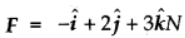Where i, j, k are unit vectors along the x-, y- and z-axis of the system respectively. What is the work done by this force in moving the body a distance of 4 m along the z-axis?

Solution :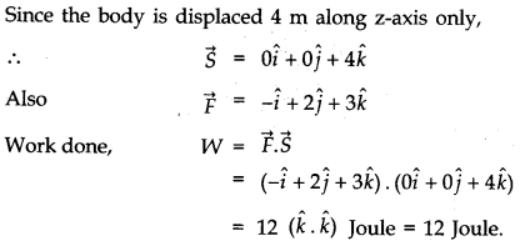Question12. An electron and a proton are detected in a cosmic ray experiment, the first with kinetic energy 10 keV, and the second with 100 keV. Which is faster, the electron or the proton? Obtain the ratio of their speeds. (electron mass = 9.11 × 10–31 kg, proton mass = 1.67 × 10–27 kg, 1 eV = 1.60 × 10–19 J).

Solution :
Electron is faster; Ratio of speeds is 13.54 : 1 Mass of the electron, me = 9.11 × 10–31 kg
Mass of the proton, mp = 1.67 × 10– 27 kg
Kinetic energy of the electron, EKe = 10 keV = 104 eV
= 104 × 1.60 × 10–19
= 1.60 × 10–15 J
Kinetic energy of the proton, EKp = 100 keV = 105 eV = 1.60 × 10–14 J
For the velocity of an electron ve, its kinetic energy is given by the relation:
EKe = (1/2) mve2
∴ ve = (2EKe / m)1/2
= (2 × 1.60 × 10-15 / 9.11 × 10-31)1/2  =  5.93 × 107 m/s
For the velocity of a proton vp, its kinetic energy is given by the relation:
EKp = (1/2)mvp2
vp = (2 × 1.6 × 10-14 / 1.67 × 10-27 )1/2  =  4.38 × 106 m/s
Hence, the electron is moving faster than the proton.
The ratio of their speeds
ve / vp = 5.93 × 107 / 4.38 × 106  =  13.54 : 1

Question13. A rain drop of radius 2 mm falls from a height of 500 m above the ground. It falls with decreasing acceleration (due to viscous resistance of the air) until at half its original height, it attains its maximum (terminal) speed, and moves with uniform speed thereafter. What is the work done by the gravitational force on the drop in the first and second half of its journey? What is the work done by the resistive force in the entire journey if its speed on reaching the ground is 10 m s–1?

Solution :
Radius of the rain drop, r = 2 mm = 2 × 10–3 m Volume of the rain drop, V = (4/3)πr3
= (4/3)  × 3.14  × (2  × 10-3)3 m-3
Density of water, ρ = 103 kg m–3
Mass of the rain drop, m = ρV
= (4/3)  × 3.14 × (2  × 10-3)3  × 103 kg
Gravitational force, F = mg
= (4/3)  × 3.14  × (2 × 10-3)3  × 103  × 9.8  N
The work done by the gravitational force on the drop in the first half of its journey:
WI = Fs
= (4/3)  × 3.14  × (2 × 10-3)3  × 103  × 9.8  × 250  =  0.082 J
This amount of work is equal to the work done by the gravitational force on the drop in the second half of its journey, i.e., WII, = 0.082 J
As per the law of conservation of energy, if no resistive force is present, then the total energy of the rain drop will remain the same.
∴Total energy at the top:
ET = mgh + 0
= (4/3) × 3.14  × (2 × 10-3)3  × 103  × 9.8  × 500  × 10-5
= 0.164 J
Due to the presence of a resistive force, the drop hits the ground with a velocity of 10 m/s.
∴Total energy at the ground:
EG = (1/2) mv2 + 0
= (1/2)  × (4/3)  × 3.14  × (2 × 10-3)3  × 103  × 9.8  × (10)2
= 1.675  × 10-3 J
∴Resistive force = EG – ET = –0.162 J

Question14. A molecule in a gas container hits a horizontal wall with speed 200 m s–1 and angle 30° with the normal, and rebounds with the same speed. Is momentum conserved in the collision? Is the collision elastic or inelastic?

Solution :
The momentum of the gas molecule remains conserved whether the collision is elastic or inelastic.
The gas molecule moves with a velocity of 200 m/s and strikes the stationary wall of the container, rebounding with the same speed.
It shows that the rebound velocity of the wall remains zero. Hence, the total kinetic energy of the molecule remains conserved during the collision. The given collision is an example of an elastic collision.

Question15. A pump on the ground floor of a building can pump up water to fill a tank of volume 30 m3 in 15 min. If the tank is 40 m above the ground, and the efficiency of the pump is 30%, how much electric power is consumed by the pump?

Solution :
Volume of the tank, V = 30 m3 Time of operation, t = 15 min = 15 × 60  = 900 s
Height of the tank, h = 40 m
Efficiency of the pump, η = 30 %
Density of water, ρ = 103 kg/m3
Mass of water, m = ρV = 30 × 103 kg
Output power can be obtained as:
P0 = Work done / Time  = mgh / t
= 30  × 103  × 9.8 ×  40 / 900  =  13.067 × 103 W
For input power Pi,, efficiency η, is given by the relation:
η = P0 / Pi  =  30%
Pi = 13.067  × 100  × 103  / 30
= 0.436  × 105 W  =  43.6 kW

Question16. Two identical ball bearings in contact with each other and resting on a frictionless table are hit head-on by another ball bearing of the same mass moving initially with a speed V. If the collision is elastic, which of the following figure is a possible result after collision?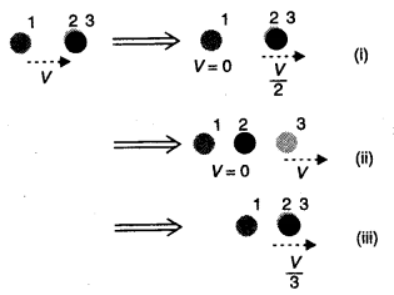Solution :
It can be observed that the total momentum before and after collision in each case is constant.
For an elastic collision, the total kinetic energy of a system remains conserved before and after collision.
For mass of each ball bearing m, we can write:
Total kinetic energy of the system before collision:
= (1/2) mV2 + (1/2) (2m)  × 02
= (1/2) mV2

Case (i)
Total kinetic energy of the system after collision:
= (1/2) m  × 0 + (1/2) (2m) (V/2)2
= (1/4)mV2
Hence, the kinetic energy of the system is not conserved in case (i).

Case (ii)
Total kinetic energy of the system after collision:
= (1/2) (2m) × 0  + (1/2) mV2
= (1/2)  mV2
Hence, the kinetic energy of the system is conserved in case (ii).

Case (iii)
Total kinetic energy of the system after collision:
= (1/2) (3m) (V/3)2
= (1/6) mV2
Hence, the kinetic energy of the system is not conserved in case (iii).

Hence, Case II is the only possibility.

Question17.The bob A of a pendulum released from 30° to the vertical hits another bob B of the same mass at rest on a table as shown in Fig. 6.15. How high does the bob A rise after the collision? Neglect the size of the bobs and assume the collision to be elastic.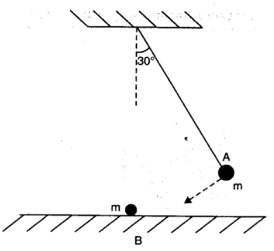Solution :
The bob A will not rise because when two bodies of same mass undergo an elastic collision, their velocities are interchanged. After collision, ball A will come to rest and the ball B would move with the velocity of A, Fig. 6.15. Thus the bob A will not rise after the collision.

Question18. he bob of a pendulum is released from a horizontal position. If the length of the pendulum is 1.5 m, what is the speed with which the bob arrives at the lowermost point, given that it dissipated 5% of its initial energy against air resistance?

Solution :
Length of the pendulum, l = 1.5 m
Mass of the bob = m
Energy dissipated = 5%
According to the law of conservation of energy, the total energy of the system remains constant.
At the horizontal position:
Potential energy of the bob, EP = mgl
Kinetic energy of the bob, EK = 0
Total energy = mgl … (i)
At the lowermost point (mean position):
Potential energy of the bob, EP = 0
Kinetic energy of the bob, EK = (1/2)mv2
Total energy Ex = (1/2)mv2    ....(ii)
As the bob moves from the horizontal position to the lowermost point, 5% of its energy gets dissipated.
The total energy at the lowermost point is equal to 95% of the total energy at the horizontal point, i.e.,
(1/2)mv2 = (95/100) mgl
∴  v = (2 × 95  × 1.5  ×  9.8 / 100)1/2
= 5.28 m/s

Question19.A trolley of mass 300 kg carrying a sandbag of 25 kg is moving uniformly with a speed of 27 km/h on a frictionless track. After a while, sand starts leaking out of a hole on the floor of the trolley at the rate of 0.05 kg s–1. What is the speed of the trolley after the entire sand bag is empty?

Solution :
As the trolley carrying the sand bag is moving uniformly, therefore, external force on the system = 0.
When the sand leaks out, it does not lead to the application of any external force on the trolley. Hence, the speed of the trolley shall not change.

Question20. A body of mass 0.5 kg travels in a straight line with velocity v = ax3/2 where a = 5 m1/2 s-1. What is the work done by the net force during its displacement from x = 0 to x = 2 m?

Solution :
Mass of the body, m = 0.5 kg Velocity of the body is governed by the equation, v =  ax3/2 and a = 5  m1/2 s-1
Initial velocity, u (at x = 0) = 0
Final velocity v (at x = 2 m) = 10√2 m/s
Work done, W = Change in kinetic energy
= (1/2) m (v2 - u2)
= (1/2)  ×  0.5 [ (10√2)2  - 02]
= (1/2)  ×  0.5 × 10  ×  10  ×  2
= 50 J

Question21. The blades of a windmill sweep out a circle of area A.

a.  If the wind flows at a velocity v perpendicular to the circle, what is the mass of the air passing through it in time t?

b.  What is the kinetic energy of the air?

c.  Assume that the windmill converts 25% of the wind’s energy into electrical energy, and that A = 30 m2, v = 36 km/h and the density of air is 1.2 kg m–3. What is the electrical power produced?

Solution :
Area of the circle swept by the windmill = A Velocity of the wind = v
Density of air = ρ
a. Volume of the wind flowing through the windmill per sec = Av
Mass of the wind flowing through the windmill per sec = ρAv
Mass m, of the wind flowing through the windmill in time t = ρAvt

b.  Kinetic energy of air = (1/2) mv2
= (1/2) (ρAvt) v2 = (1/2) ρAv3t

c. Area of the circle swept by the windmill = A = 30 m2
Velocity of the wind = v =  36 km/h
Density of air,  ρ = 1.2 kg m–3
Electric energy produced = 25% of the wind energy
= (25/100)  ×  Kinetic energy of air
= (1/8)  ρ A v3t
Electrical power = Electrical energy / Time
= (1/8)  ρ A v3t / t
= (1/8)  ρ A v3
= (1/8)  ×  1.2 × 30  ×  (10)3
= 4.5 kW

Question22. A person trying to lose weight (dieter) lifts a 10 kg mass, one thousand times, to a height of 0.5 m each time. Assume that the potential energy lost each time she lowers the mass is dissipated.

a. How much work does she do against the gravitational force?

b.  Fat supplies 3.8 × 107 J of energy per kilogram which is converted to mechanical energy with a 20% efficiency rate. How much fat will the dieter use up?

Solution :
a.  Mass of the weight, m = 10 kg Height to which the person lifts the weight, h = 0.5 m
Number of times the weight is lifted, n = 1000
∴Work done against gravitational force:
= n(mgh)
= 1000  ×  10  ×  9.8  ×  0.5  =  49 kJ

b.   Energy equivalent of 1 kg of fat = 3.8  × 107 J
Efficiency rate = 20%
Mechanical energy supplied by the person’s body:
= (20/100)  × 3.8 × 107 J
= (1/5)  × 3.8  × 107 J
Equivalent mass of fat lost by the dieter:
= [ 1 / (1/5)  ×  3.8 × 107 ] ×  49 × 103
= (245 / 3.8) × 10-4 = 6.45  ×  10-3 kg

Question23. A family uses 8 kW of power.

a.  Direct solar energy is incident on the horizontal surface at an average rate of 200 W per square meter. If 20% of this energy can be converted to useful electrical energy, how large an area is needed to supply 8 kW?

b.  Compare this area to that of the roof of a typical house.

Solution :
(a) Power used by the family, P = 8 kW = 8 × 103 W Solar energy received per square metre = 200 W
Efficiency of conversion from solar to electricity energy = 20 %
Area required to generate the desired electricity = A
As per the information given in the question, we have:
8 × 103 = 20% × (A × 200)
= (20 /100) × A × 200
∴ A = 8 × 103 / 40  =  200 m2

(b) The area of a solar plate required to generate 8 kW of electricity is almost equivalent to the area of the roof of a building having dimensions 14 m × 14 m. (≈ √200).

Question24.A bullet of mass 0.012 kg and horizontal speed 70 m s–1 strikes a block of wood of mass 0.4 kg and instantly comes to rest with respect to the block. The block is suspended from the ceiling by means of thin wires. Calculate the height to which the block rises. Also, estimate the amount of heat produced in the block.

Solution :
Mass of the bullet, m = 0.012 kg Initial speed of the bullet, ub = 70 m/s
Mass of the wooden block, M = 0.4 kg
Initial speed of the wooden block, uB = 0
Final speed of the system of the bullet and the block = ν
Applying the law of conservation of momentum:
mub + MuB = (m + M) v
0.012 × 70 + 0.4 × 0 = (0.012 + 0.4) v
∴ v = 0.84 / 0.412 = 2.04 m/s
For the system of the bullet and the wooden block:
Mass of the system, m' = 0.412 kg
Velocity of the system = 2.04 m/s
Height up to which the system rises = h
Applying the law of conservation of energy to this system:
Potential energy at the highest point = Kinetic energy at the lowest point
m'gh = (1/2)m'v2
∴ h = (1/2)(v2 / g)
= (1/2)  ×  (2.04)2 / 9.8
= 0.2123 m
The wooden block will rise to a height of 0.2123 m.
Heat produced = Kinetic energy of the bullet – Kinetic energy of the system
= (1/2) mu2 - (1/2) m'v2 = (1/2)  ×  0.012  ×  (70)2 - (1/2)  ×  0.412  ×  (2.04)2
= 29.4 -  0.857 = 28.54 J

Question25.Two inclined frictionless tracks, one gradual and the other steep meet at A from where two stones are allowed to slide down from rest, one on each track (Fig. 6.16). Will the stones reach the bottom at the same time? Will they reach there with the same speed? Explain. Given θ1 = 30°, θ2 = 60°, and h = 10 m, what are the speeds and times taken by the two stones?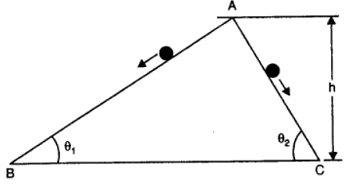Solution :
AB and AC are two smooth planes inclined to the horizontal at ∠θ1 and ∠θ2 respectively. As height of both the planes is the same, therefore, noth the stones will reach the bottom with same speed.
As P.E. at O = K.E. at A = K.E. at B
∴ mgh = 1/2 mv12 = 1/2 mv22
∴ v1 = v2
As it is clear from fig. above, accleration of the two blocks are a1 = g sin θ1 and a2 = g sin θ2
As θ2 > θ1
∴ a2 > a1
From v = u + at = 0 + at
or, t = v/a
As t ∝ 1/a, and a2 > a1
∴ t2 < t1
i.e., Second stone will take lesser time and reach the bottom earlier than the first stone.

Question26.A 1 kg block situated on a rough incline is connected to a spring of spring constant 100 N m–1 as shown in Fig. 6.17. The block is released from rest with the spring in the unstretched position. The block moves 10 cm down the incline before coming to rest. Find the coefficient of friction between the block and the incline. Assume that the spring has a negligible mass and the pulley is frictionless.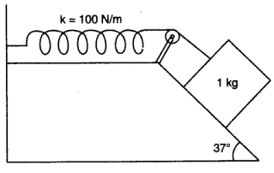Solution :
Mass of the block, m = 1 kg
Spring constant, k = 100 N m–1
Displacement in the block, x = 10 cm = 0.1 m
The given situation can be shown as in the following figure.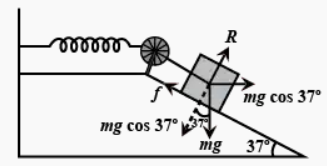At equilibrium: Normal reaction, R = mg cos 37°
Frictional force, f = μ R = mg Sin 370
Where, μ is the coefficient of friction
Net force acting on the block  = mg sin 37° – f
= mgsin 37° – μmgcos 37°
= mg(sin 37° – μcos 37°)
At equilibrium, the work done by the block is equal to the potential energy of the spring, i.e.,
mg(sin 37° – μcos 37°)x =  (1/2)kx2
1 × 9.8 (Sin 370 - μcos 37°) = (1/2)  × 100 × (0.1)
0.602 - μ  ×  0.799 = 0.510
∴ μ = 0.092  / 0.799  =  0.115

Question27.A bolt of mass 0.3 kg falls from the ceiling of an elevator moving down with an uniform speed of 7 m s–1. It hits the floor of the elevator (length of the elevator = 3 m) and does not rebound. What is the heat produced by the impact? Would your answer be different if the elevator were stationary?

Solution :
Mass of the bolt, m = 0.3 kg
Speed of the elevator = 7 m/s
Height, h = 3 m
Since the relative velocity of the bolt with respect to the lift is zero, at the time of impact, potential energy gets converted into heat energy.
Heat produced = Loss of potential energy
= mgh = 0.3 × 9.8 × 3
= 8.82 J
The heat produced will remain the same even if the lift is stationary. This is because of the fact that the relative velocity of the bolt with respect to the lift will remain zero.

Question28.A trolley of mass 200 kg moves with a uniform speed of 36 km/h on a frictionless track. A child of mass 20 kg runs on the trolley from one end to the other (10 m away) with a speed of 4 m s–1 relative to the trolley in a direction opposite to the its motion, and jumps out of the trolley. What is the final speed of the trolley? How much has the trolley moved from the time the child begins to run?

Solution :
Mass of the trolley, M = 200 kg
Speed of the trolley, v = 36 km/h = 10 m/s
Mass of the boy, m = 20 kg
Initial momentum of the system of the boy and the trolley
= (M + m)v
= (200 + 20) × 10
= 2200 kg m/s
Let v' be the final velocity of the trolley with respect to the ground.
Final velocity of the boy with respect to the ground = v' - 4
Final momentum = Mv' + m(v' - 4)
= 200v' + 20v' - 80
= 220v' – 80
As per the law of conservation of momentum:
Initial momentum = Final momentum
2200 = 220v' – 80
∴ v' = 2280 / 220 = 10.36 m/s
Length of the trolley, l = 10 m
Speed of the boy, v'' = 4 m/s
Time taken by the boy to run, t = 10/4 = 2.5 s
∴ Distance moved by the trolley = v'' × t = 10.36 × 2.5 = 25.9 m

Question29. Which of the following potential energy curves in Fig. 6.18 cannot possibly describe the elastic collision of two billiard balls? Here r is the distance between centres of the balls.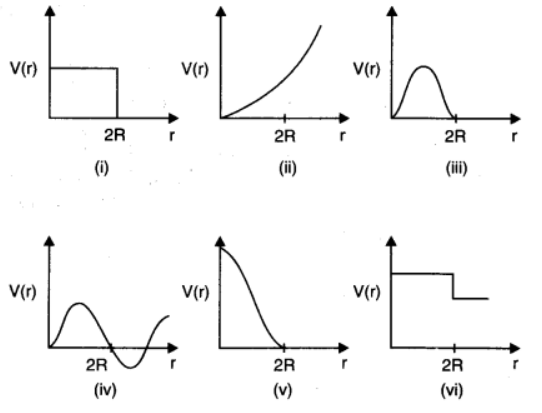Solution : The potential energy of a system of two masses is inversely proportional to the separation between them. In the given case, the potential energy of the system of the two balls will decrease as they come closer to each other. It will become zero (i.e., V(r) = 0) when the two balls touch each other, i.e., at r = 2R, where R is the radius of each billiard ball. The potential energy curves given in figures (i), (ii), (iii), (iv), and (vi) do not satisfy these two conditions. Hence, they do not describe the elastic collisions between them.

Question30.Show that the two-body decay of this type must necessarily give an electron of fixed energy and, therefore, cannot account for the observed continuous energy distribution in the β-decay of a neutron or a nucleus (Fig. 6.19).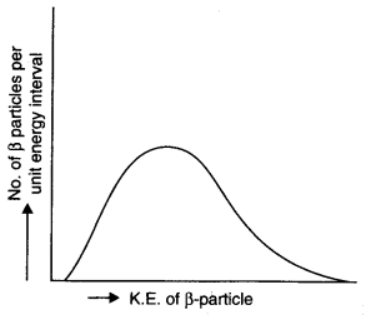Note: The simple result of this exercise was one among the several arguments advanced by W. Pauli to predict the existence of a third particle in the decay products of β-decay. This particle is known as neutrino. We now know that it is a particle of intrinsic spin ½ (like e, p or n), but is neutral, and either massless or having an extremely small mass (compared to the mass of electron) and which interacts very weakly with matter. The correct decay process of neutron is: n → p + e+ ν]

Solution :
The decay process of free neutron at rest is given as:
n → p + e
From Einstein’s mass-energy relation, we have the energy of electron as Δmc2
Where,
Δm = Mass defect = Mass of neutron – (Mass of proton + Mass of electron)
c = Speed of light

Δm and c are constants. Hence, the given two-body decay is unable to explain the continuous energy distribution in the β-decay of a neutron or a nucleus. The presence of neutrino νon the LHS of the decay correctly explains the continuous energy distribution.